This is a paper by Ross and Brian which attempts to put mate selection into a useful framework for crossbreeding scenarios. It aims to generate both investment matings (to make eg. F1 females) as well as matings to capitalise on investments (to make eg. 3-breed crosses with F1 dams) in competition with each other.

Computational problems are such that Group Mate Selection will be needed for implementation.

A TACTICAL APPROACH TO

THE DESIGN OF CROSSBREEDING PROGRAMS.

R.K. Shepherd1 and B.P. Kinghorn2

1 Department of Mathematics and Computing, University of Central Queensland,

Rockhampton, Qld 4702, Australia

2 Department of Animal Science, University of New England, Armidale, NSW 2351, Australia

ABSTRACT

A Look Ahead Mate Selection (LAMS) scheme is developed with the objective of maximising phenotypic merit in each of the next two generations. This is of most value where non-additive effects are of importance, such as heterosis, inbreeding or non-additive QTL effects. The method is developed on the basis of a crossbreeding scenario, but it can be applied to any situation for which appropriate merit functions can be derived. Earlier work aimed just two generations ahead, but this can be suboptimal for non-additive effects, as ‘investment matings’ (such as generation of first cross females) are not properly exploited, due to lack of incentive to generate merit in the following generation. The method developed is tactical, in that it uses prevailing animal resources and generates a mate selection solution which constitutes the breeding decisions for the current mating. Integer programming and genetic algorithms can be used to find optimal solutions, but animal grouping strategies may be required to give realistic computing times.

INTRODUCTION

A tactical approach to making decisions about breeding for economic merit involves two steps:

• Develop an objective function which describes net economic merit in terms of selection and mating decisions
• Develop and implement a mate selection algorithm which maximises the objective function

The paper focuses on use of this approach when breed differences and heterosis are important, in addition to genetic variation within groups.

AIMING ONE OR TWO GENERATIONS AHEAD

If the objective is to maximise progeny genetic merit then mate selection can be carried out using Linear Programming (LP) techniques (Jansen and Wilton, 1985) or an approximate method (Kinghorn, 1986) which is less demanding computationally. Kinghorn and Shepherd (1994) extended the breeding horizon by aiming two generations ahead in order to maximise the genetic merit of the grandprogeny. The intention was to give due consideration to the exploitation of assortative mating and to enrich information in the pedigree structure by rearing relatives in different fixed effects groups, such as herd or management group ie. to breed for connection. An exchange algorithm was used to implement mate selection.

Aiming two generations ahead is very important for crossbreeding, most especially where maternal heterosis is important. For example, the optimum commercial animals two generations ahead may be the product of a three breed cross resulting from a terminal sire mated to an F1 female which is produced from matings in the current generation. Similarly a four breed cross can be aimed at two generations ahead by producing the two breed crosses from the current matings.

However, it may not be optimal to determine mate selection in each generation solely on the basis of maximising the predicted genetic merit in the grandprogeny. For example, consider a crossbreeding scenario without any within-breed additive genetic variation. If a three breed cross is optimum two generations ahead, the mating algorithm will set up F1 females in the next generation. However next generation when these animals are available for breeding, the mate selection algorithm may not select them because aiming two generations ahead the alternative mating to produce F1 females in the next generation may be optimal. The problem results from not distinguishing between matings to produce breeding animals (investment matings) and matings to produce commercial offspring (realisations of prior investments). This problem can be largely overcome by simultaneously considering merit both one and two generations ahead.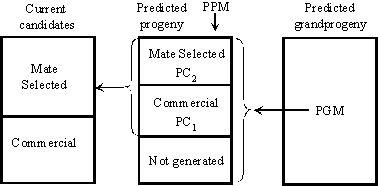Figure 1.

AIMING BOTH ONE AND TWO GENERATIONS AHEAD

Figure 1 illustrates a Look Ahead Mate Selection (LAMS) scheme which carries out mate selection among predicted progeny, and, as a consequence mate selection among current candidates. Only commercial animals yield dollar returns from product each generation, and this is used to calculate predicted progeny merit (PPM) and predicted grandprogeny merit (PGM). The breeding objective is to maximise the combined return from commercial animals both one and two generations in the future. A full consideration would accommodate discounting of returns, which will not be done here, for simplicity. Some progeny (denoted by PC1) are predicted to be commercial animals in the next generation. Other progeny (denoted by PC2) are predicted to produce commercial animals two generations ahead, and are thus mate selected. In general, most predicted progeny are not generated, as the generation 0 mate selection set does not include their parents. Mate selection among predicted progeny also drives the mate selection of the current candidates, which is used to drive the breeding program.

Each generation, predicted progeny roles (PPR, which are either PC2 or PC1, or not generated) are determined by maximising the objective function. It should be emphasised that PC1 and PC2 are not sets of current generation mating pairs but rather sets of predicted progeny with predicted roles in later generations. A candidate pair for mating in the current generation may have some progeny predicted to be in PC1, and other progeny predicted to be in PC2. Each generation, PPR are determined according to predicted progeny merit (PPM), predicted grandprogeny merit (PGM), and the outcome of the optimisation algorithm, described below. This algorithm finds the mating set for the current generation which maximises the objective function, based on PPR and appropriate constraints according to the logistics of mating.

PREDICTED PROGENY AND GRANDPROGENY MERIT

To calculate PPM and PGM, we need genetic (and economic) models that account appropriately for sources of variation. Consider a crossbreeding scenario in which the only important sources of variation are within breed additive genetic variation (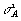) and assumed known fixed between-breed effects: direct additive (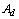), maternal additive (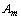), direct dominance (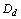) and maternal dominance (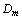) effects. The predicted progeny merit (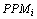) is calculated as follows: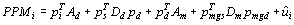(1)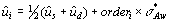(2)

• where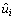,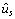and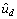are the EBVs of predicted progeny i and its sire and dam,
• where the vector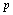represents the proportion of genes of each breed in either the individual (i), sire (s), dam (d), maternal grandsire (mgs) or maternal granddam (mgd),
• where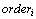is the order statistic for individual i which characterises the deterministic Mendelian sampling for each sex,
• and where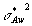is the sum of two variances; one due to within family additive genetic variation [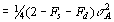] and the other due to the error variance of the predicted family mean [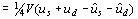]. The part due to prediction error variance is necessary as predicted progeny will be selected for future roles and thus less accurately evaluated family means will provide more progeny variation. Note that this sets up appropriate reward for the increased future selection opportunity given by choosing less accurately evaluated animals. This is especially valuable under overlapping generations (Goddard and Howarth, 1994).

Using the same notation, the predicted grandprogeny merit (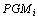) is calculated as follows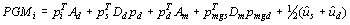(3)

where no within family variation is needed as no selection will be applied. If epistatis is known to be important in explaining non-additive genetic variation between breeds and good estimates exist then it should be included in PPM and PGM. For example, additive x additive epistatic interaction would involve adding the term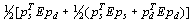to equations (1) and (2) where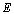is the matrix of between breed additive x additive epistatic effects - for example, E = (J - I)e assuming equality of breed epistatic effects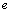, the maximum possible breakdown of favourable purebreed epistatic effects.

MATE SELECTION ALGORITHM

Each pair of predicted progeny can have any of three roles: not generated (ie. the parents are not mated), generated for PC1, or generated and selected for PC2. Now the role value of the kth predicted male progeny of the mating between candidates i and j and the rth predicted female progeny of the mating between candidates p and q is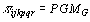for PC2 where G is the offspring of the kth predicted male and rth predicted female, while for PC1 the role value is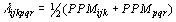.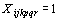(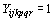) if the predicted progeny pair is selected for a PC2 (PC1) role, otherwise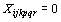(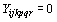). Deciding the role of a predicted progeny pair is not straightforward, as it depends on the allocated roles of other predicted progeny.

Let n be the number of progeny of each sex per dam and assume at most d dams per sire. If x mating pairs are required to be selected in each generation then the problem can be stated formally as follows, where PP is predicted progeny:

Maximise: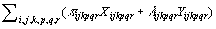subject to

No. of matings: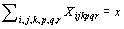(4)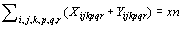(5)

Individual PP: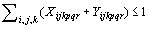for each p, q, r (6)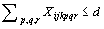for each i, j, k (7)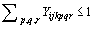for each i, j, k (8)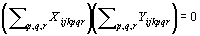for each i, j, k (9)

Full sib PP: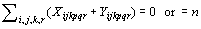for each p, q (10)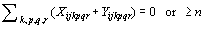for each i, j (11)

Half sib PP: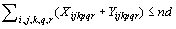for each p (12)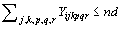for each i (13)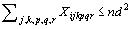for each i (14)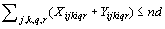for each i (15)

The constraint equations can be interpreted as follows:

Eq. 4. There are exactly x PC2 matings. This constraint can be lifted, with the consequence that the emphasis on genetic merit in generations one and two will differ.

Eq. 5. There are exactly xn PP pairs selected for PC (PC1 and PC2) roles.

Eq. 6. Each female PP can be selected for at most one role.

Eq. 7. Each male PP can be allocated at most d mates for PC2.

Eq. 8. Each male PP can be selected for at most one PC1 role.

Eq. 9. Each male PP can be selected for a role as either PC1 or PC2 but not both.

Eq. 10. Full sib female PP either are not generated (= 0) or are selected for PC roles (= n).

Eq. 11. Full sib male PP either are not generated (= 0) or are selected for at least n PC roles.

Eq. 12. For male candidate p there are at most d matings, each generating n female PP.

Eq. 13. For male candidate i there are at most d matings, each generating n male PP who could be allocated PC1 roles.

Eq. 14. For male candidate i there are at most d matings, each generating n male PP, with each able to be used in at most d PC2 roles.

Eq. 15. For male candidate i there are at most d half sib families with all n female PP selected for PC roles

To put some emphasis on controlling inbreeding, further constraints could be added. For example, to not allow any predicted full sib progeny to be mated, add the constraint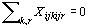for each i, j combination. Alternatively, a proper modelling of inbreeding depression in PPM and PGM will give mate selection to reduce inbreeding coefficients in the next two generations.

An LP could solve this problem, except for constraints (9), (10) and (11). Constraint (9) would vanish for single pair mating (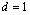), if aiming only one generation ahead (only PC1) or if aiming only two generations ahead (only PC2). The (or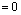) option in constraints (10) and (11) could be removed by only considering PC role allocation, rather than including PP which are not generated. That is, given a particular parental mating set, find the best allocation of roles to predicted progeny in order to maximise the objective.

However, even as an LP, the problem is still too large. For example, with 1000 (m) males and 1000 (f) females available for selection and 1 (n) progeny of each sex per dam then the total number of possible predicted progeny is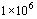(mfn) and there would be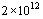(2 x mfn x mfn) unknown binary variables in the matrices X and Y. The algorithm of Kinghorn (1986) would have great computational efficiency here. Hence in general the task is an integer nonlinear programming (NLP) problem which is not computationally feasible for typical crossbreeding schemes. Good approximate methods are required to solve this mate selection problem.

EVOLUTIONARY ALGORITHMS AND GROUP MATE SELECTION

Genetic Algorithms, GA’s, and the more recent Evolutionary Algorithms, EA’s (Michalewicz, 1994) mimic biological evolution in their quest to find the best solution for a complex optimisation problem. The earlier NLP implicitly selects between all possible matings sets which is usually a very large number (see box below). However many of these mating sets are not very competitive for the objective. Hence an alternative approach would be to use an EA to search among competitive mating sets in generation 0. Then for each mating set evaluated, the NLP problem becomes one of mate selection in generation 1. For a particular generation 0 mating set the number of predicted progeny reduces to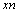per sex and the number of elements in the matrices X and Y reduces to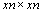, a much smaller NLP problem. For example, for m = f = 1000, n = 1 and x = 1000 the number of elements reduces to. An EA could be designed to solve the mate selection problem directly. However it is probably more time efficient to use an EA to search among possible mating sets in conjunction with a different EA designed to solve the generation 1 mate selection problem for each mating set. Hayes et al (1998) discuss the application of genetic and evolutionary algorithms to the mate selection problem.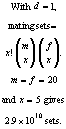One approach to reducing computation time is to group animals and to run a mate selection algorithm at the level of groups, leading to a solution describing the number of animals to be selected out of each group and the pattern of mate allocation (Kinghorn, 1998). This can be followed by a ‘fine tuning’ individual animal mate selection using only animals selected out of groups. This 2-step approach gave high efficiency yet dramatically lower computing times (Kinghorn, 1998).

EXAMPLE

The example relates to a tiny problem for which there is one male and one female candidate of each of breeds 1 and 2 in generation 0. Two predicted progeny of each sex (n = 2) are generated for each of the four candidate matings – eg. males 11a and 11b, and females 11c and 11d from the mating 1 x 1 (male x female). Figure 2 illustrates all possible matings among these predicted progeny.

Table 1 shows the PPM for predicted progeny and PGM for their crosses. For simplicity of presentation in this table, matrix l is replaced by a column of PPM values for males and a row of PPM values for females. This leads to an alternative set of constraints, sufficient for this example and described in the caption of Table 1. For two single pair matings to be selected in each of generations 0 and 1 (x = 2, d = 1), one optimal solution is matings 1 x 2 and 2 x 1 in generation 0, matings 12b x 21d and 21b x 12d in generation 1, and PC1 roles for progeny 12a and 12c.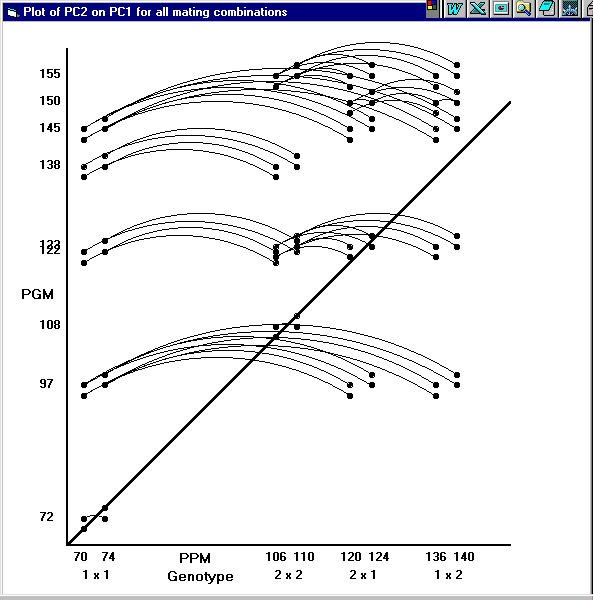Figure 2. Plot of PGM (predicted grandprogeny merit) on PPM (predicted progeny merit) for the example. All possible individual matings between predicted progeny are shown. Groups of four points at the diagonal represent matings within purebreeds (and within full sib families in this tiny example). Matings exactly on the diagonal are between predicted progeny of equal breeding value, and those just off the diagonal are between predicted progeny of unequal breeding value. All matings between predicted progeny of different PPM are linked by an arc. PGM are generally higher than PPM because, in this example, maternal heterosis is only expressed in generation 2.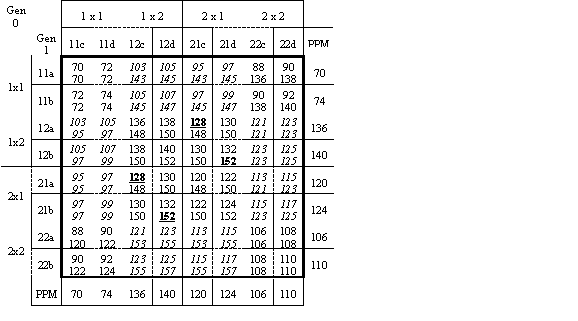Table 1. Average PPM (top within cell) and PGM (bottom within cell) both within the bold frame, for the example. Male (female) predicted progeny are listed in the second column (row). PPM values are also shown in the right (male) and bottom (female) margins. Ad1=80, Ad2=100, Am1=-8, Am2=8, Dd=40, Dm=40. Predicted progeny a and c (b and d) have breeding values of +2 (-2) as deviations from breed and family means. Given d=1, constraints on solutions (0 or 1 for each location within each cell) are: each generation 0 row plus matched column S =0 or S =2n, each generation 1 row or column S £ 1, and (optionally, see comment on eq.4) total PC2 S =x. Cells in italics are not feasible under single-pair mating. A solution for x= 2 matings per generation is shown in bold and underlined.

DISCUSSION

As presented in this paper, each predicted progeny pair is chosen on the basis of either PPM or PGM - analogous to use of independent culling levels (ICL). This will not be generally optimal. For example, consider two PP pairs, both with PGM > PPM, with one pair marginally superior in PGM but markedly inferior in PPM. The pair with marginally lower PGM should be selected, given the potential prospect of PC1 roles for these animals. This is especially true if the accuracy of genetic parameters to estimate PPM and PGM is not high. However, using a simple index of PPM and PGM as the criterion of merit, rather than ICL, will miss the power that this approach has for setting up matings specifically targeted either one or two generations ahead. One possible solution is to use a composite index which is a weighted average of ICL and a simple index. A related issue arises when product can be harvested from breeding animals, in which case PC2 matings contribute product in the next generation.

With proper specification and modelling of the objective function, the method presented here has prospects of being able to exploit non-additive QTL effects, exploit assortative mating, avoid inbreeding depression one and two generations ahead, and set up a 4-pathway progeny testing scheme and various MOET schemes (where flexibility to choose fecundity is modelled).

The present method aims to maximise the sum of merit in commercial offspring in generations 1 and 2 alone. It ignores later generations, which is a weakness. For example, increasing the breeding values of a pair of predicted progeny has an equal impact on their average PPM and on the PGM if they were to be mated. This means that the ‘breeding line’ has no more power to attract high EBV animals than the ‘commercial line’. This could be overcome by extending the objective function to give appropriate weighting to long-term genetic merit, and other factors such as inbreeding level. Relative weightings over time will differ between factors. For example, there might be short-term emphasis on expression of heterosis and favourable QTL genotypes, but longer term emphasis on polygenic breeding value and optimal QTL allele frequencies.

ACKNOWLEDGEMENTS

Much of this work was conducted during a visit by the authors to the Roslin Institute and the Scottish Agricultural College, Edinburgh, with funds provided by the Australian DIST and the UK BBSRC Underwood Fund.

REFERENCES

Jansen, G.B. and Wilton, J.W. (1985) J. Dairy Sc. 68:1302-1305

Goddard, M.E. and Howarth, J.M. (1995) Proc. 5th WCGALP 18:306-309

Hayes, B.J., Shepherd, R.K., Newman, S. and Kinghorn, B.P. (1998) Proc. 6th WCGALP

Kinghorn, B.P. (1986) Proc. 3rd WCGALP 12:233-244

Kinghorn, B.P. (1998) J. Dairy. Science. Submitted.

Kinghorn, B.P. and Shepherd, R.K. (1990) Proc. 4th WCGALP 15:7-15

Kinghorn, B.P. and Shepherd, R.K. (1994) Proc. 5th WCGALP 18:255-261

Michalewicz, Z (1994) Genetic algorithms + data structures = evolution programs 2nd edition, Springer-Verlag, Berlin.